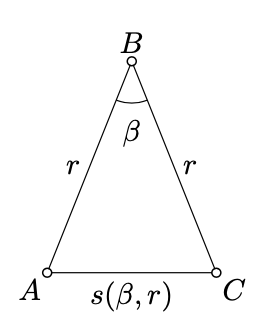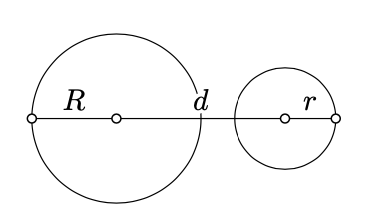$$\newcommand{\id}{\mathrm{id}}$$ $$\newcommand{\Span}{\mathrm{span}}$$ $$\newcommand{\kernel}{\mathrm{null}\,}$$ $$\newcommand{\range}{\mathrm{range}\,}$$ $$\newcommand{\RealPart}{\mathrm{Re}}$$ $$\newcommand{\ImaginaryPart}{\mathrm{Im}}$$ $$\newcommand{\Argument}{\mathrm{Arg}}$$ $$\newcommand{\norm}{\| #1 \|}$$ $$\newcommand{\inner}{\langle #1, #2 \rangle}$$ $$\newcommand{\Span}{\mathrm{span}}$$

# 3.5: Triangle with the given sides

$$\newcommand{\vecs}{\overset { \rightharpoonup} {\mathbf{#1}} }$$ $$\newcommand{\vecd}{\overset{-\!-\!\rightharpoonup}{\vphantom{a}\smash {#1}}}$$$$\newcommand{\id}{\mathrm{id}}$$ $$\newcommand{\Span}{\mathrm{span}}$$ $$\newcommand{\kernel}{\mathrm{null}\,}$$ $$\newcommand{\range}{\mathrm{range}\,}$$ $$\newcommand{\RealPart}{\mathrm{Re}}$$ $$\newcommand{\ImaginaryPart}{\mathrm{Im}}$$ $$\newcommand{\Argument}{\mathrm{Arg}}$$ $$\newcommand{\norm}{\| #1 \|}$$ $$\newcommand{\inner}{\langle #1, #2 \rangle}$$ $$\newcommand{\Span}{\mathrm{span}}$$ $$\newcommand{\id}{\mathrm{id}}$$ $$\newcommand{\Span}{\mathrm{span}}$$ $$\newcommand{\kernel}{\mathrm{null}\,}$$ $$\newcommand{\range}{\mathrm{range}\,}$$ $$\newcommand{\RealPart}{\mathrm{Re}}$$ $$\newcommand{\ImaginaryPart}{\mathrm{Im}}$$ $$\newcommand{\Argument}{\mathrm{Arg}}$$ $$\newcommand{\norm}{\| #1 \|}$$ $$\newcommand{\inner}{\langle #1, #2 \rangle}$$ $$\newcommand{\Span}{\mathrm{span}}$$

Consider the triangle $$ABC$$. Set

$$a = BC$$,                     $$b = CA$$,                     $$c = AB.$$

Without loss of generality, we may assume that

$$a \le b \le c.$$

Then all three triangle inequalities for $$\triangle ABC$$ hold if and only if

$$c \le a + b$$.

The following theorem states that this is the only restriction on $$a$$, $$b$$, and $$c$$.

Theorem $$\PageIndex{1}$$

Assume that $$0 < a \le b \le c \le a + b$$. Then there is a triangle with sides $$a, b$$, and $$c$$; that is, there is $$\triangle ABC$$ such that $$a = BC$$, $$b = CA$$, and $$c = AB$$.

Proof

Fix the points $$A$$ and $$B$$ such that $$AB = c$$. Given $$\beta \in [0, \pi]$$, suppose that $$C_{\beta}$$ denotes the point in the plane such that $$BC_{\beta} = a$$ and $$\measuredangle ABC = \beta$$.

According the Corollary $$\PageIndex{1}$$, the map $$\beta \mapsto C_{\beta}$$ is continuous. Therefore, the function $$b(\beta) = AC_{\beta}$$is continous (formally, it follows from Exercise 1.9.1 and Exercise 1.9.2).

Note that $$b(0) = c - a$$ and $$b (\pi) = c + a$$. Since $$c - a \le b \le c + a$$, by the intermediate value theorem (Theorem 3.2.1) there is $$\beta_0 \in [0, \pi]$$ such that $$b(\beta_0) = b$$, hence the result.Assume $$r > 0$$ and $$\pi > \beta > 0$$. Consider the triangle $$ABC$$ such that $$AB = BC = r$$ and $$\measuredangle ABC = \beta$$. The existence of such a triangle follows from Axiom IIIa and Proposition 2.2.2.

Note that according to Axiom IV, the values $$\beta$$ and $$r$$ define the triangle $$ABC$$ up to the congruence. In particular, the distance $$AC$$ depends only on $$\beta$$ and $$r$$.

$s(\beta, r):= AC.$

Proposition $$\PageIndex{1}$$

Given $$r > 0$$ and $$\varepsilon > 0$$, there is $$\delta > 0$$ such that

$$0 < \beta < \delta \Rightarrow s(r, \beta) < \varepsilon.$$

Proof

Fix two points $$A$$ and $$B$$ such that $$AB = r$$.Choose a point $$X$$ such that $$AY = \dfrac{\varepsilon}{2}$$; it exists by Proposition 2.2.2.

Note that $$X$$ and $$Y$$ lie on the same side of $$(AB)$$; therefore, $$\angle ABY$$ is positive. Set $$\delta = \measuredangle ABY$$.

Suppose $$0 < \beta < \delta$$; by Axiom IIIa, we can choose $$B$$ so that $$\measuredangle ABC = \beta$$ and $$BC = r$$. Further we can choose a half-line $$[BZ)$$ such that $$\measuredangle ABZ = \dfrac{1}{2} \cdot \beta$$.

Note that $$A$$ and $$Y$$ lie on opposite sides of $$(BZ)$$ and moreover $$\measuredangle ABZ \equiv - \measuredangle CBZ$$. In particular, $$(BZ)$$ intersects $$[AY]$$; denote by $$D$$ the point of intersection.

Since $$D$$ lies between $$A$$ and $$Y$$, we have that $$AD < AY$$.

Since $$D$$ lies on $$(BZ)$$ we have that $$\measuredangle ABD \equiv \measuredangle CBD$$. By Axiom IV, $$\triangle ABD \cong \triangle CBD$$. It follows that

$\begin{array} {rcl} {s(r, \beta)} & = & {AC \le} \\ {} & \le & {AD + DC =} \\ {} & = & {2 \cdot AD <} \\ {} & < & {2 \cdot AY =} \\ {} & = & {\varepsilon} \end{array}$

Corollary $$\PageIndex{1}$$

Fix a real number $$r > 0$$ and two distinct points $$A$$ and $$B$$. Then for any real number $$\beta \in [0, \pi]$$, there is a unique point $$C_{\beta}$$ such that $$BC_{\beta} = r$$ and $$\measuredangle ABC_{\beta} = \beta$$. Moreover, $$\beta \mapsto C_{\beta}$$ is a continuous map from $$[0, \pi]$$ to the plane.

Proof

The existence and uniqueness of $$C_{\beta}$$ follows from Axiom IIIa and Proposition 2.2.2.

Note taht if $$\beta_1 \ne \beta_2$$, then

$C_{\beta_1} C_{\beta_2} = s(r, |\beta_1 - \beta_2|).$

By Proposition $$\PageIndex{1}$$, the map $$\beta \mapsto C_{\beta}$$ is continuous.

Given a positive real number $$r$$ and a pint $$O$$, the set $$\Gamma$$ of all points on distance $$r$$ from $$O$$ is called a circle with radius $$r$$ and center $$O$$.

Exercise $$\PageIndex{1}$$

Show that two circles intersect if and only if

$|R - r| \le d \le R + r,$

where $$R$$ and $$r$$ denote their radiuses, and $$d$$ — the distance between their centers.Hint

The "only-if" part follows from the triangle inequality. To prove "if" part, observe that Theorem $$\PageIndex{1}$$ implies existence of a triangle with sides $$r_1$$, $$r_2$$, and $$d$$. Use this triangle to show that there is a point $$X$$ such that $$O_1X = r_1$$ and $$O_2X = r_2$$, where $$O_1$$ and $$O_2$$ are the centers of the corresponding circles.